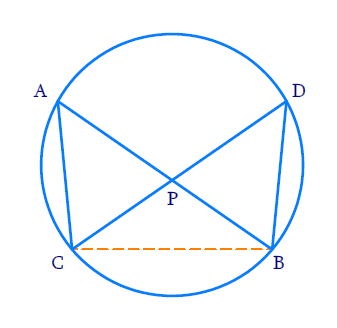# Ex. 6.6 Q7 Triangles Solution - NCERT Maths Class 10

Go back to  'Ex.6.6'

## Question

In Fig. below, two chords $$AB$$ and $$CD$$ intersect each other at the point $$P.$$

Prove that:

(i) $$\Delta APC\,\text{~}\Delta {\text{ }}DPB$$

(ii) $$AP. PB = CP. DP$$## Text Solution

Reasoning:

As we know that, two triangles, are similar if:

(i) Their corresponding angles are equal.

(ii) Their corresponding sides are in the same ratio.

As we know that angles in the same segment of a circle are equal.

Steps:

(i) In, $$\Delta APC \;\text{and}\; \Delta DPB$$

$$\angle APC = \angle DPB$$

(Vertically opposite angles)

$$\angle PAC = \angle PDB$$

(Angles in the same segment)

$$\Rightarrow \Delta APC\text{~}\Delta DPB$$

(A.A criterion)

(ii) In, $$\Delta APC \;\text{and}\; \Delta DPB$$

\begin{align} \frac{{AP}}{{PD}}& = \frac{{PC}}{{PB}} = \frac{{AC}}{{DB}} \\ & \quad \left[ {\Delta APC\text{~}\Delta DPB} \right]\\ \frac{{AP}}{{PD}} &= \frac{{PC}}{{PB}} \end{align}

$$\Rightarrow \;\;AP.PB = PC.PD$$

Learn from the best math teachers and top your exams

• Live one on one classroom and doubt clearing
• Practice worksheets in and after class for conceptual clarity
• Personalized curriculum to keep up with school